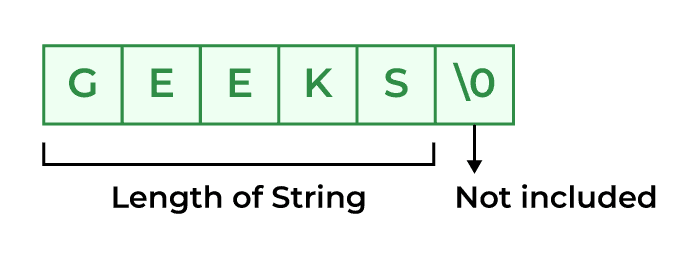# strlen() function in c

The strlen() function in C calculates the length of a given string. The strlen() function is defined in string.h header file. It doesn’t count the null character ‘\0’.

## Syntax of C strlen()

The syntax of strlen() function in C is as follows:

`size_t strlen(const char* str);`

### Parameters

The strlen() function only takes a single parameter.

• str: It represents the string variable whose length we have to find.

### Return Value

• This function returns the integral length of the string passed.C strlen() Function

## Example of C strlen()

The below programs illustrate the strlen() function in C:

## C

 `// c program to demonstrate` `// example of strlen() function.` `#include ` `#include `   `int` `main()` `{` `    ``// defining string` `    ``char` `str[] = ``"GeeksforGeeks"``;`   `    ``// getting length of str using strlen()` `    ``int` `length = ``strlen``(str);` `    ``printf``(``"Length of string is : %d"``, length);`   `    ``return` `0;` `}`

Output

`Length of string is : 13`